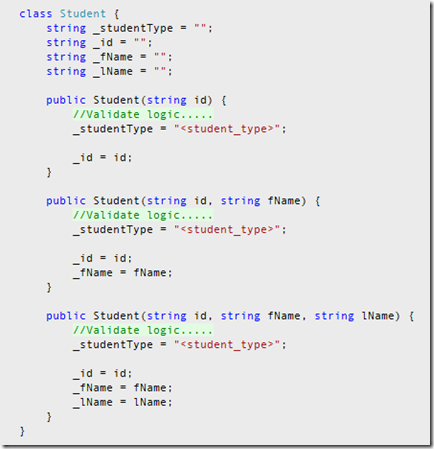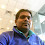## Thursday, 9 December 2010

### Constructor Chaining in C#

What’s ‘Constructor Chaining’ ?

Constructor Chaining is an approach where a constructor calls another constructor in the same or base class.

This is very handy when we have a class that defines multiple constructors. Assume we are developing a class call ‘Student’. And this class consist of 3 constructors. On each constructer we have to validate the students id and categorize him/her. So if we do not use the constructor chaining approach, it would be something similar to the one shown below:Even the above approach solve our problem, it duplicate our code. (We are assigning a value to ‘_id’ in all constructors). This where constructor chaining is very useful. It will eliminate this problem. This time we only assign values only in the constructor which consist most number of parameters. And we call that constructor, when other two constructers are called.

`class Student {        string _studentType = "";        string _id = "";        string _fName = "";        string _lName = "";        public Student(string id)            : this(id, "", "") {        }        public Student(string id, string fName)            : this(id, fName, "") {        }        public Student(string id, string fName, string lName) {            //Validate logic.....            _studentType = "<student_type>";            _id = id;            _fName = fName;            _lName = lName;        }    }`

**Please note: If you do not specify anything [In this example we used ‘this’), it will be consider that we are calling the constructor on the base class. And it’s similar to using ‘: base(…)’]

1.But how do you do constructor chaining with a different object than string or simple types?

E.g: Two Student constructors, one taking no arguments and the other takes an object Person as inparameter. How would the constructors look like?

2.Person Class
------------

class Person
{
public string FristName { get; set; }
public string LastName { get; set; }
public int Age { get; set; }

}

Student Class
-------------

class Student
{
Person _person = null;

public Student(Person person)
{
this._person = person;
}

public Student()
{

}
}

Usage
-----

Student s1 = new Student();

Person p = new Person();
p.FristName = "John";
p.LastName = "Doe";
p.Age = 28;

Student s2 = new Student(p);

----------------------------------------------

Were you referring to something like this ??